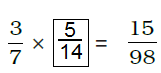# NCERT Exemplar Solutions for Class 7 Maths Chapter 2 Fractions and Decimals

NCERT Exemplar Solutions for Class 7 Maths Chapter 2 Fractions and Decimals is the best study material for those students who are finding difficulties in solving problems. These solutions can help students clear doubts quickly and help in understanding the topic effectively. Our expert tutors formulate these exercises to assist you with your exam preparation to attain good marks in the subject. Students who wish to score good marks in Maths subject, practise NCERT Exemplar Solutions for Class 7 Maths.

A fraction simply tells us how many parts of a whole we have. You can represent a fraction by the slash that is written in between the two numbers. The top number is called the numerator and the bottom number is called the denominator. A decimal is defined as a fraction, whose denominator is a power of ten and whose numerator is expressed by figures placed to the right of a decimal point. NCERT Exemplar Solutions for Class 7 Maths Chapter 2 – Fractions and Decimals PDF are available here. Now, let us have a look at the topics discussed in this Chapter.

• Addition, Subtraction, Division and Multiplication of Fractions
• Multiplication of a Fraction by a Whole Number
• Division of Whole Number by a Fraction
• Reciprocal of Fraction
• Division of a Fraction by a Whole Number
• Multiplication and Division of Decimal Numbers

## Download the PDF of NCERT Exemplar Solutions for Class 7 Maths Chapter 2 Fractions and Decimals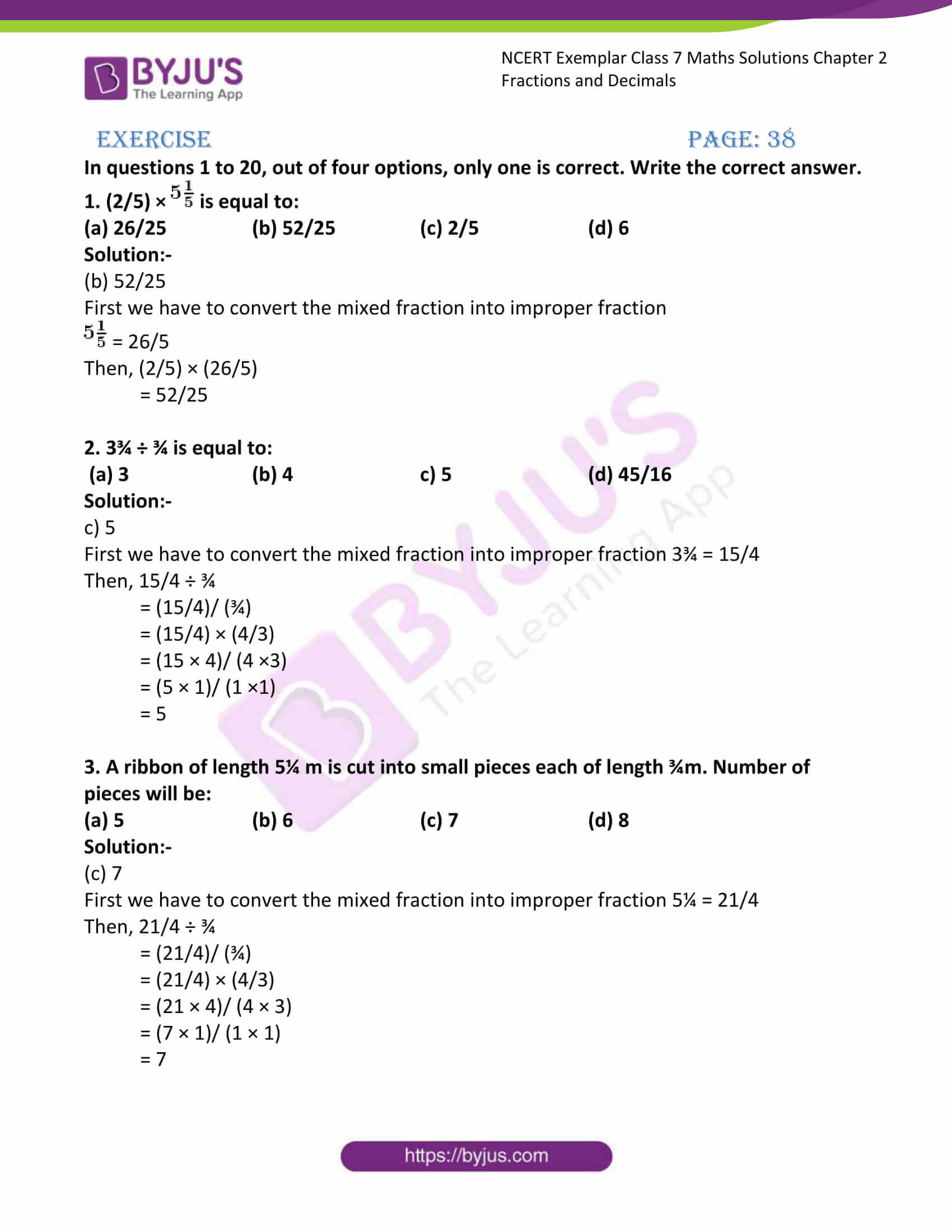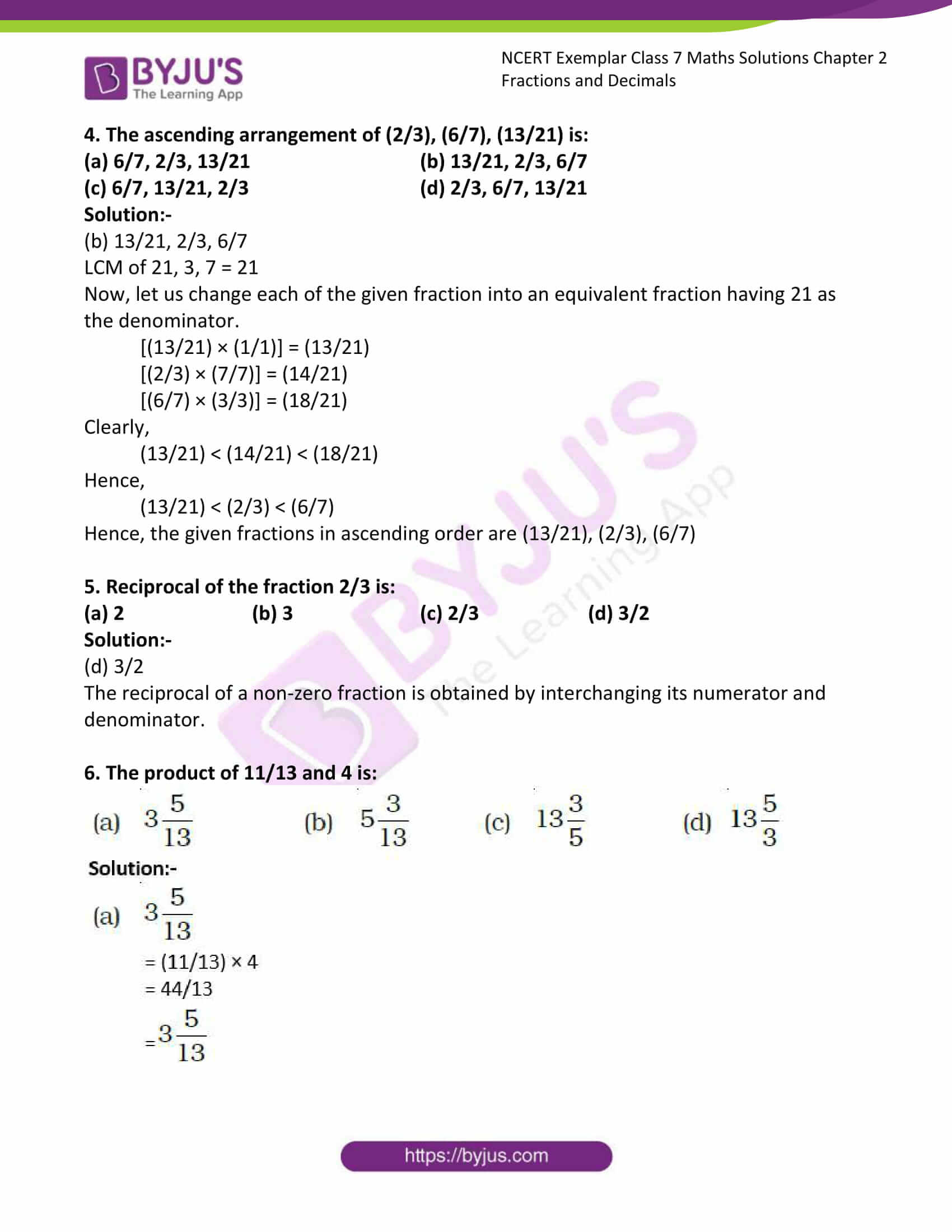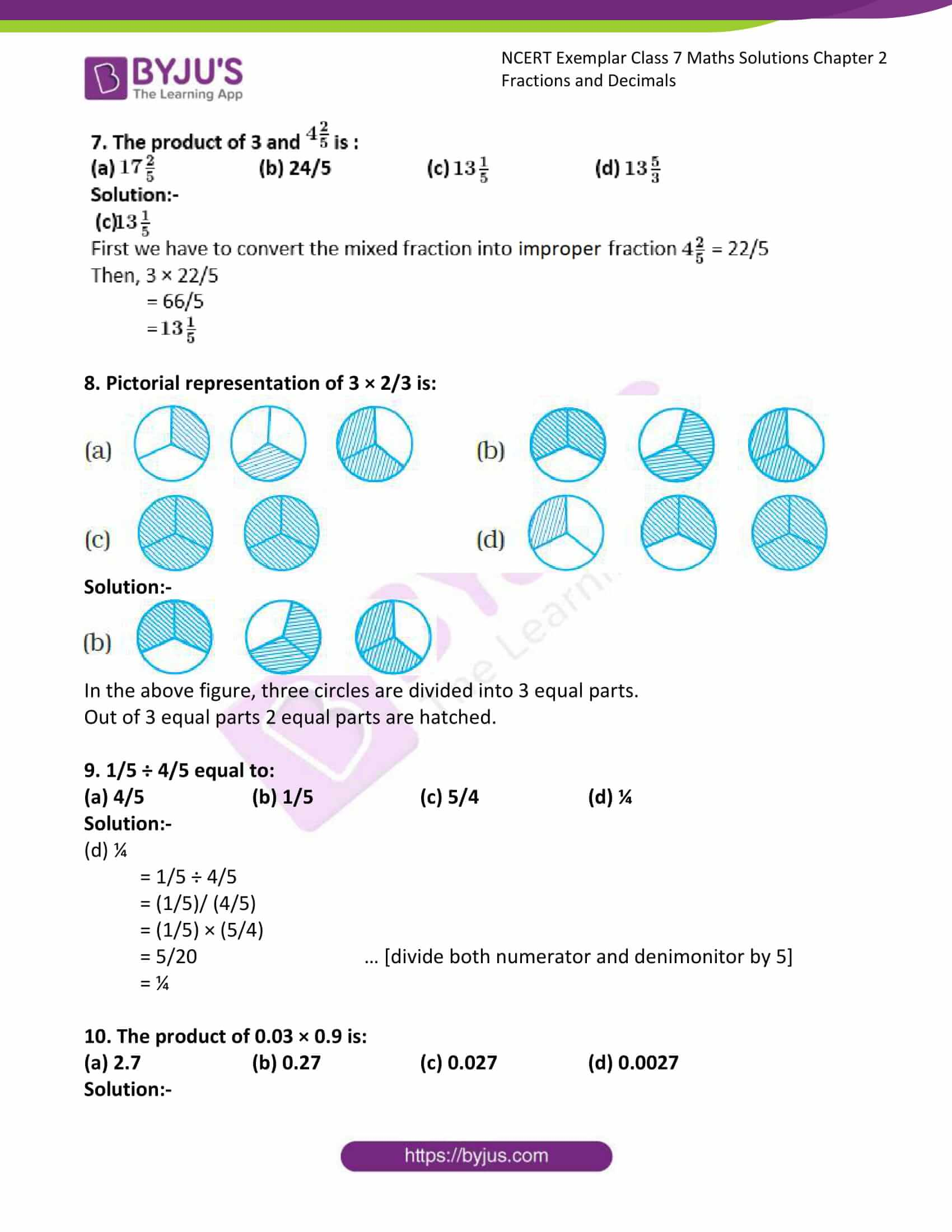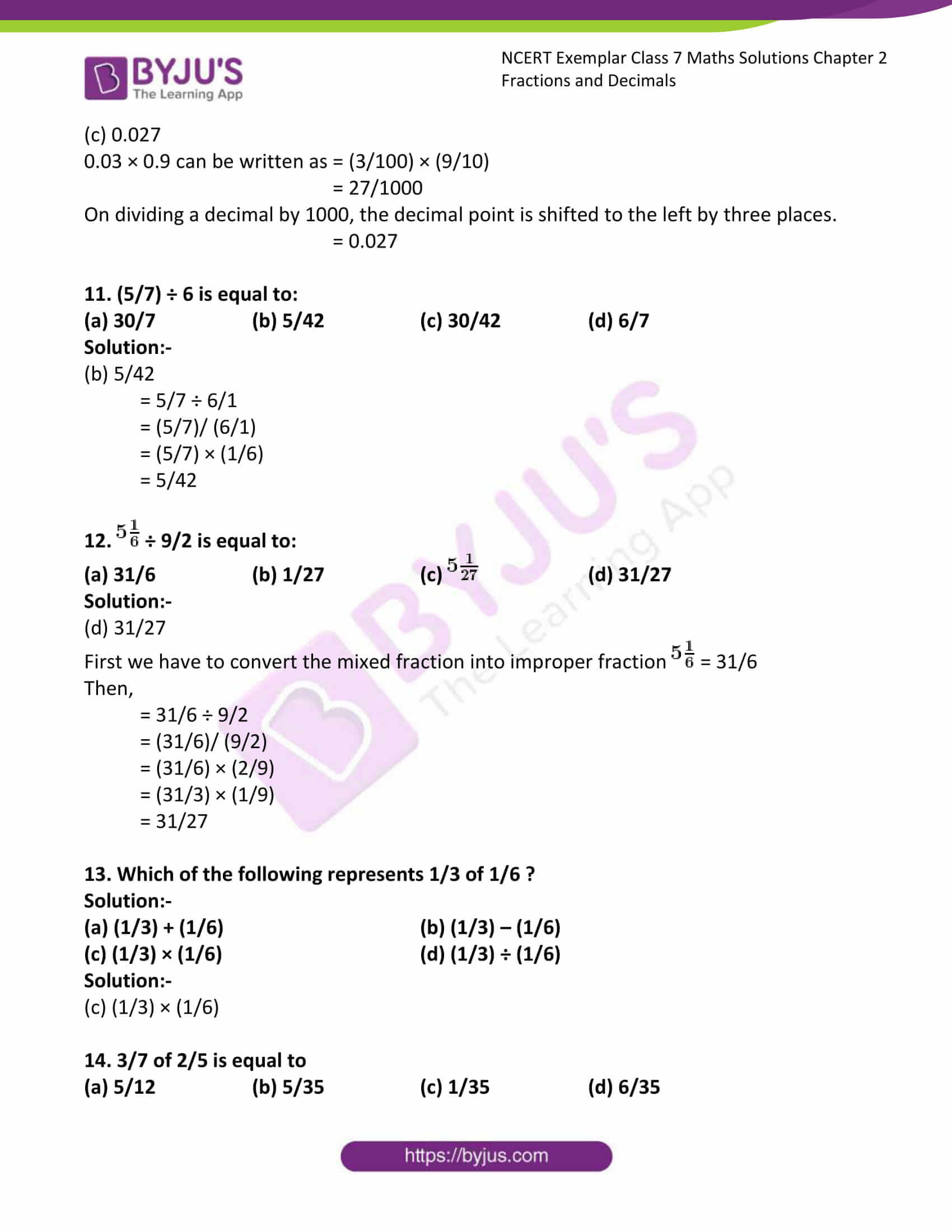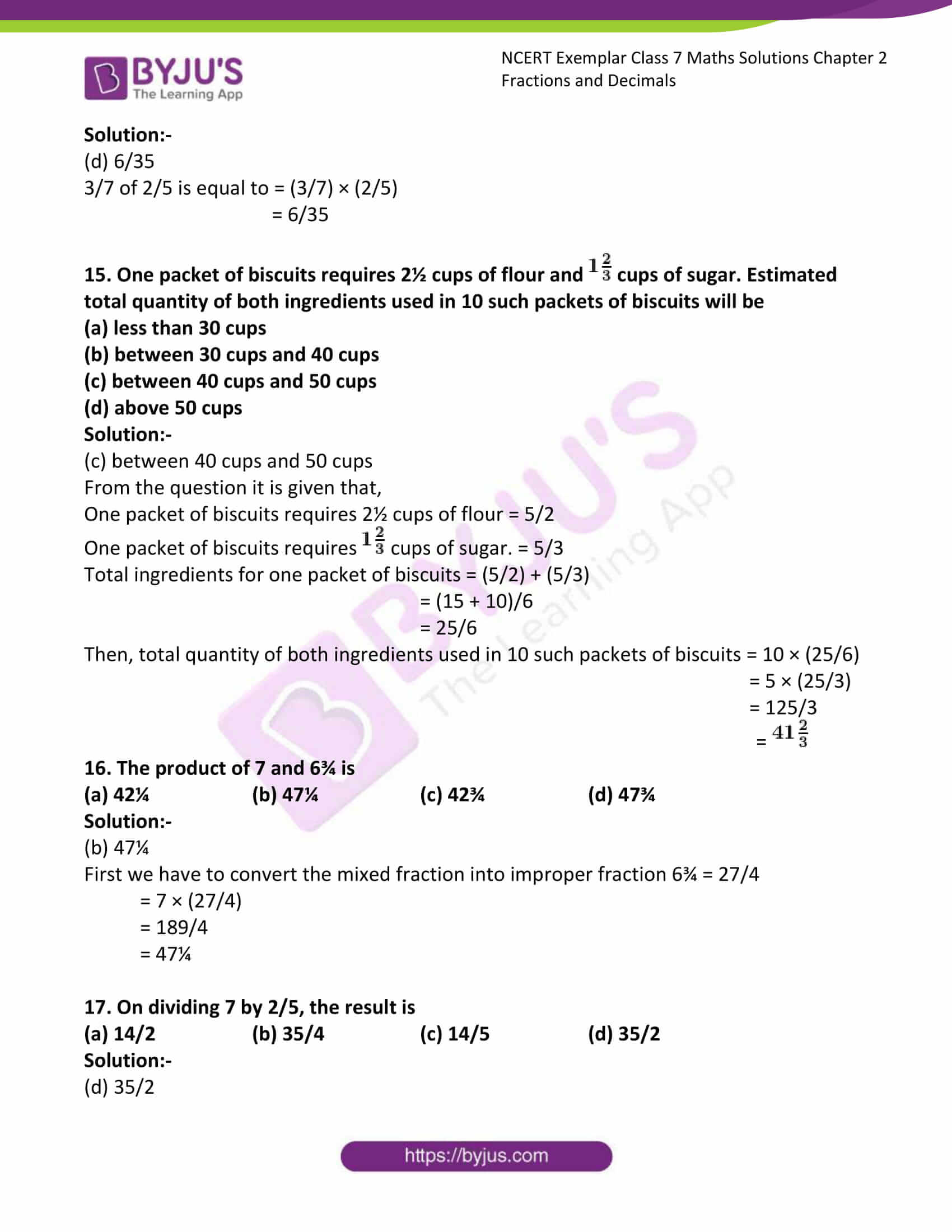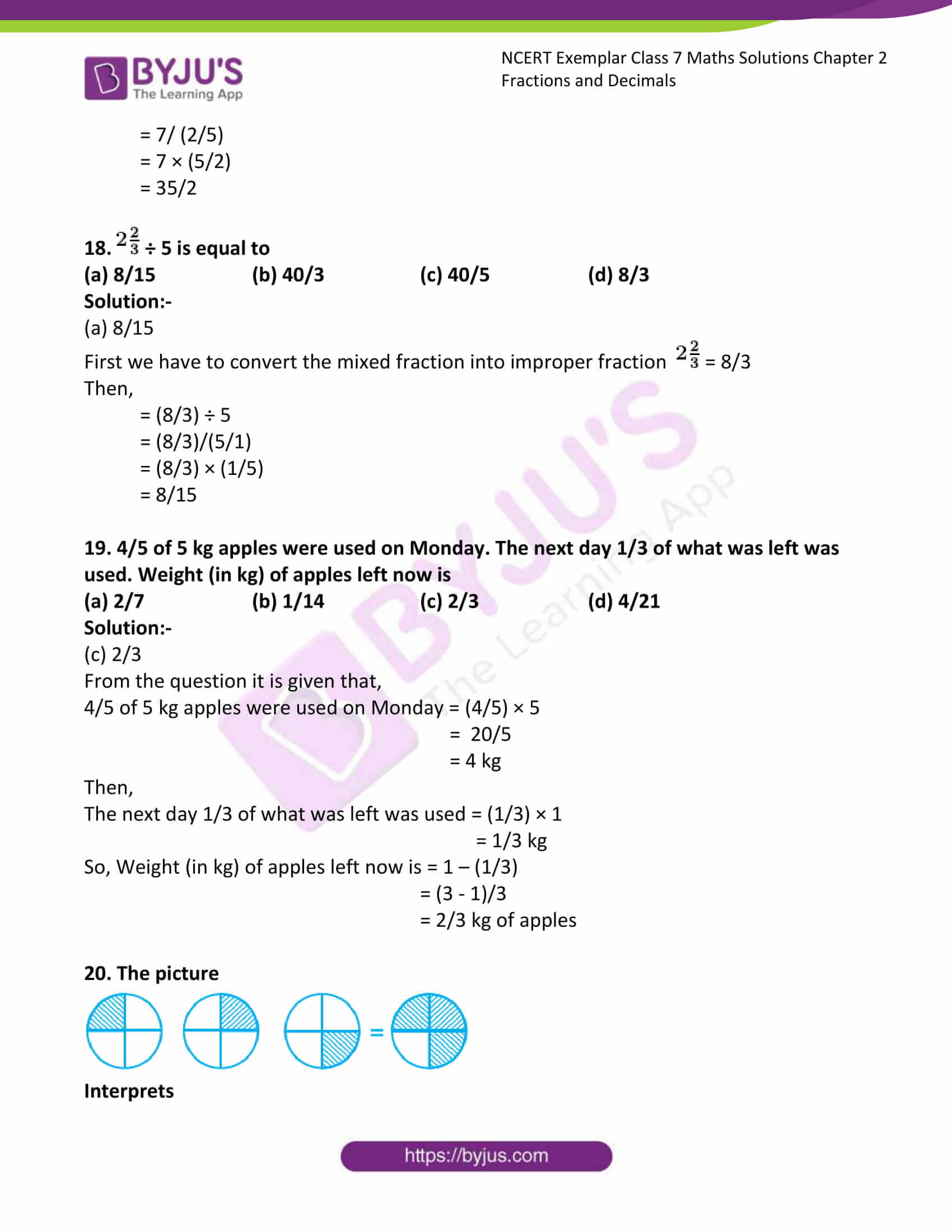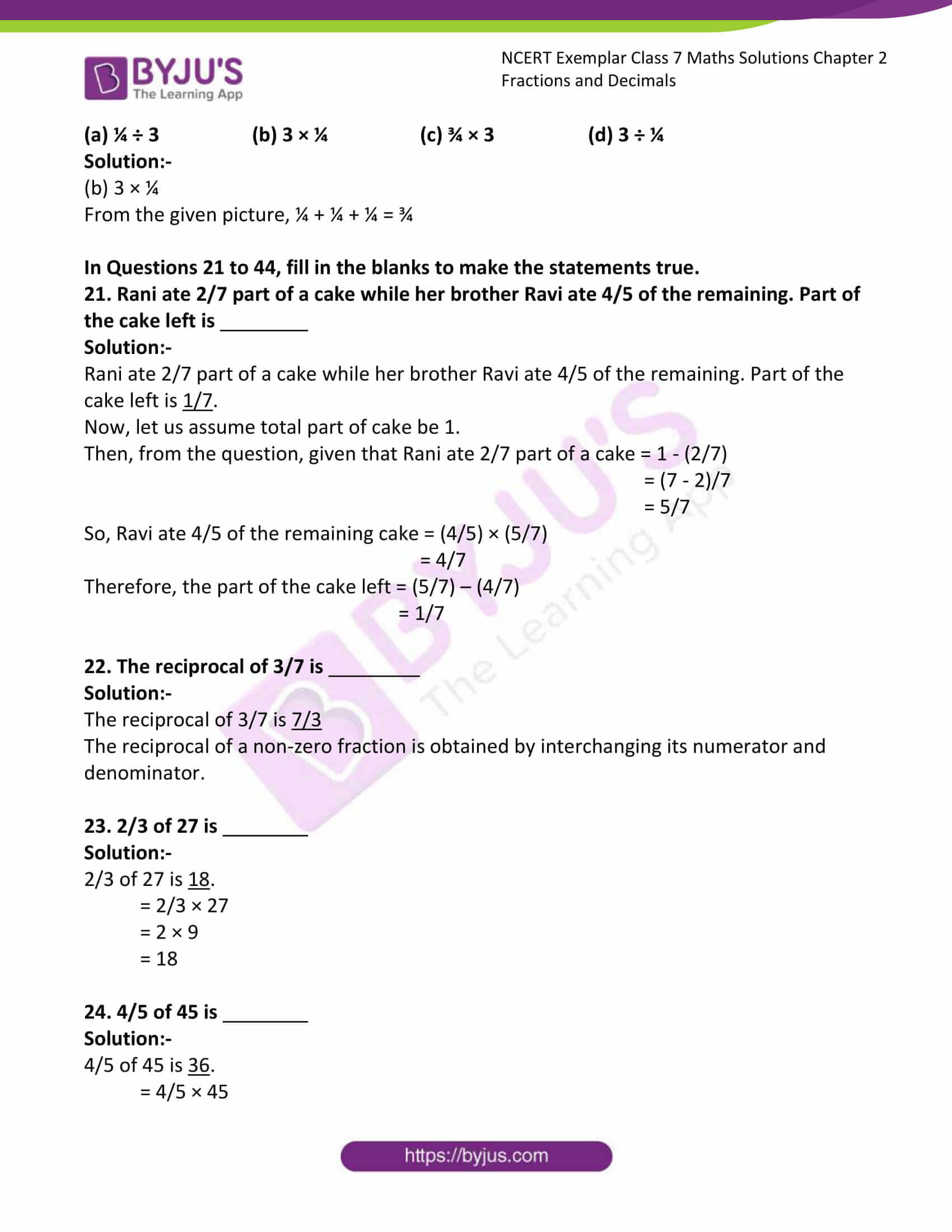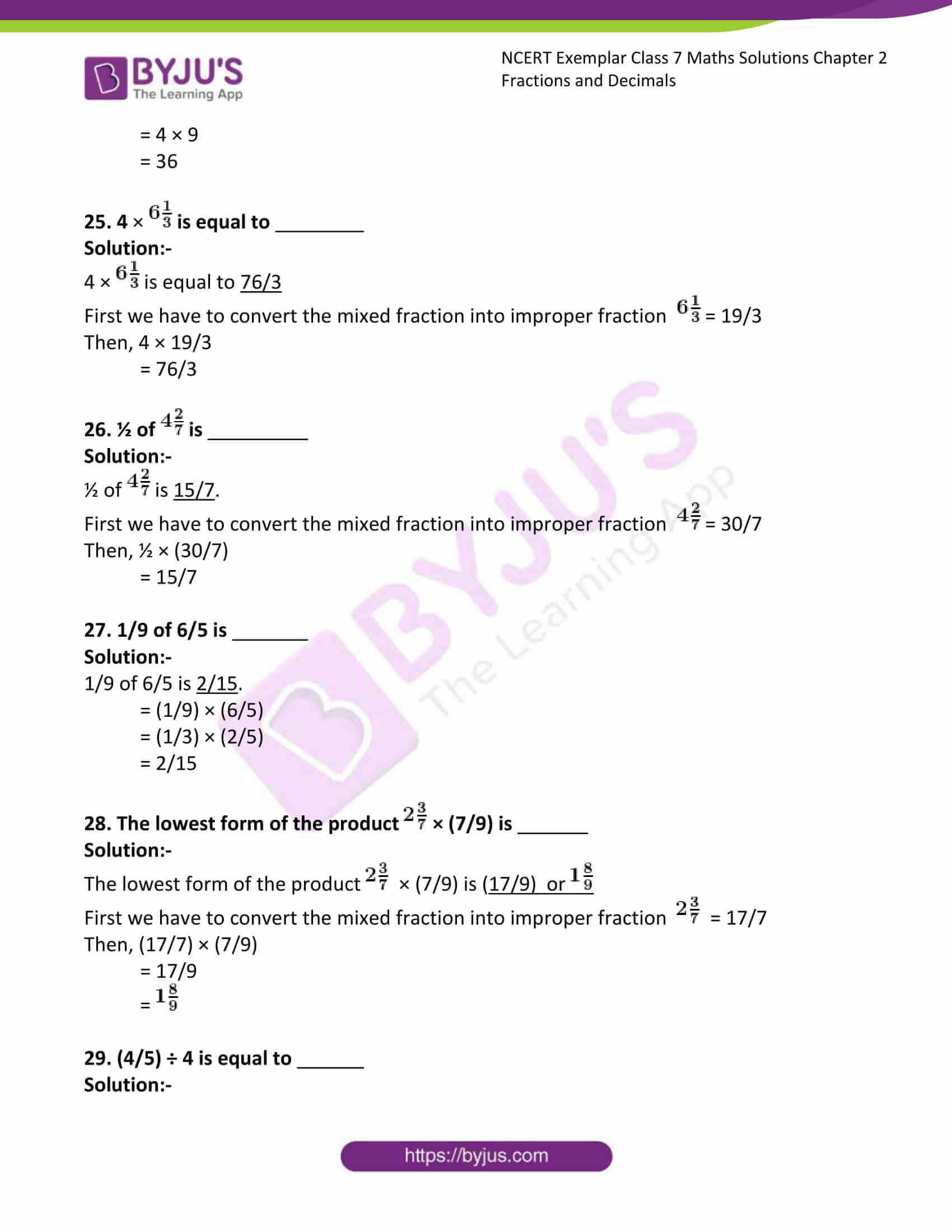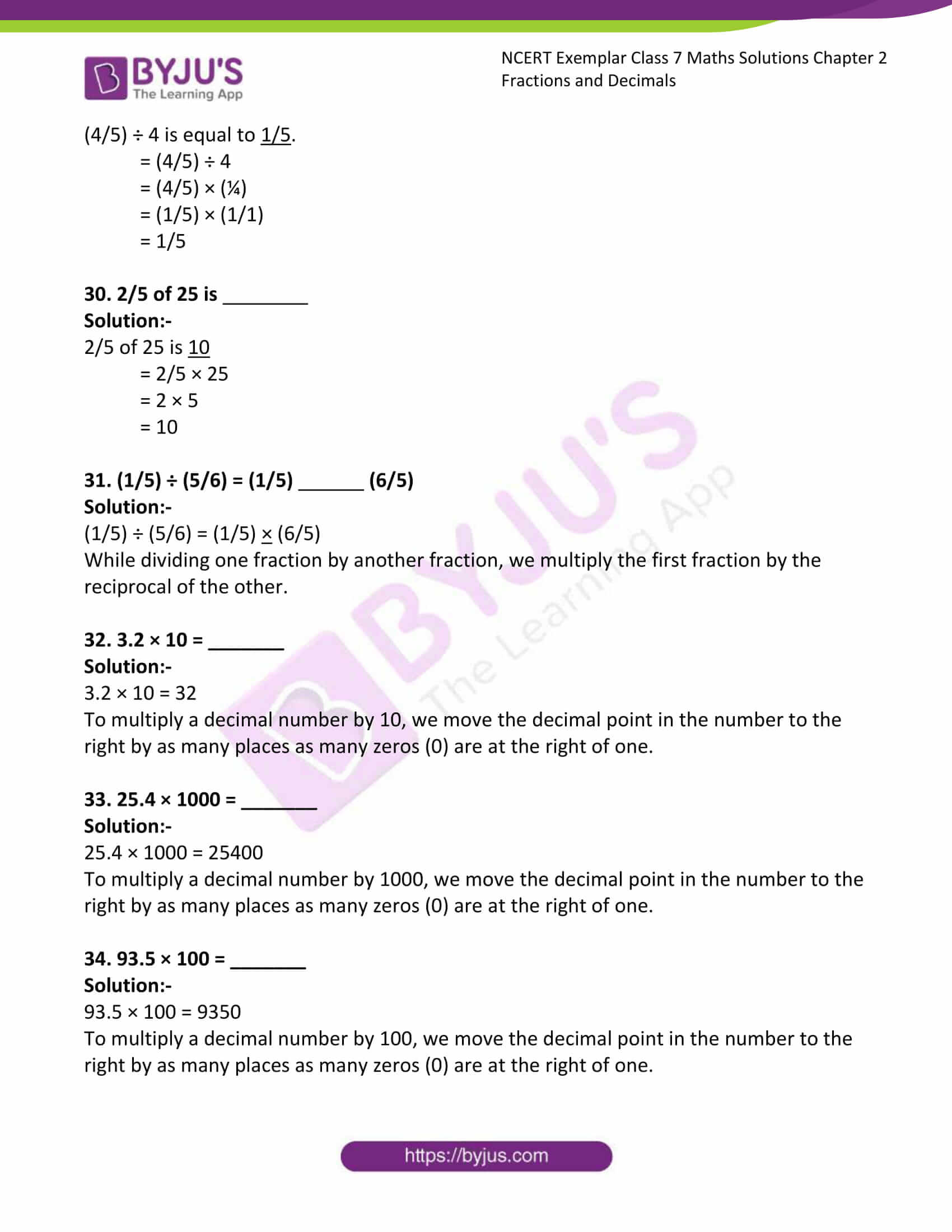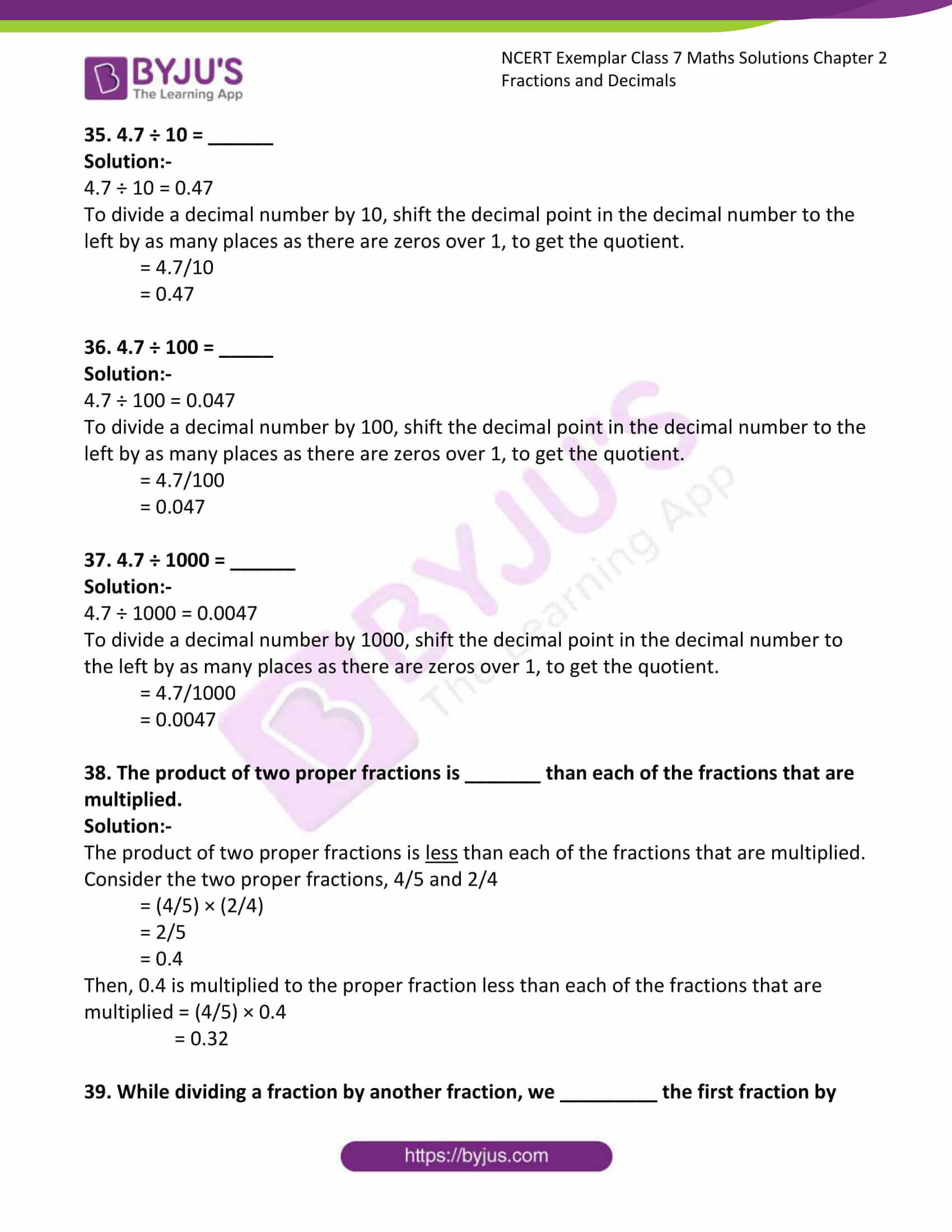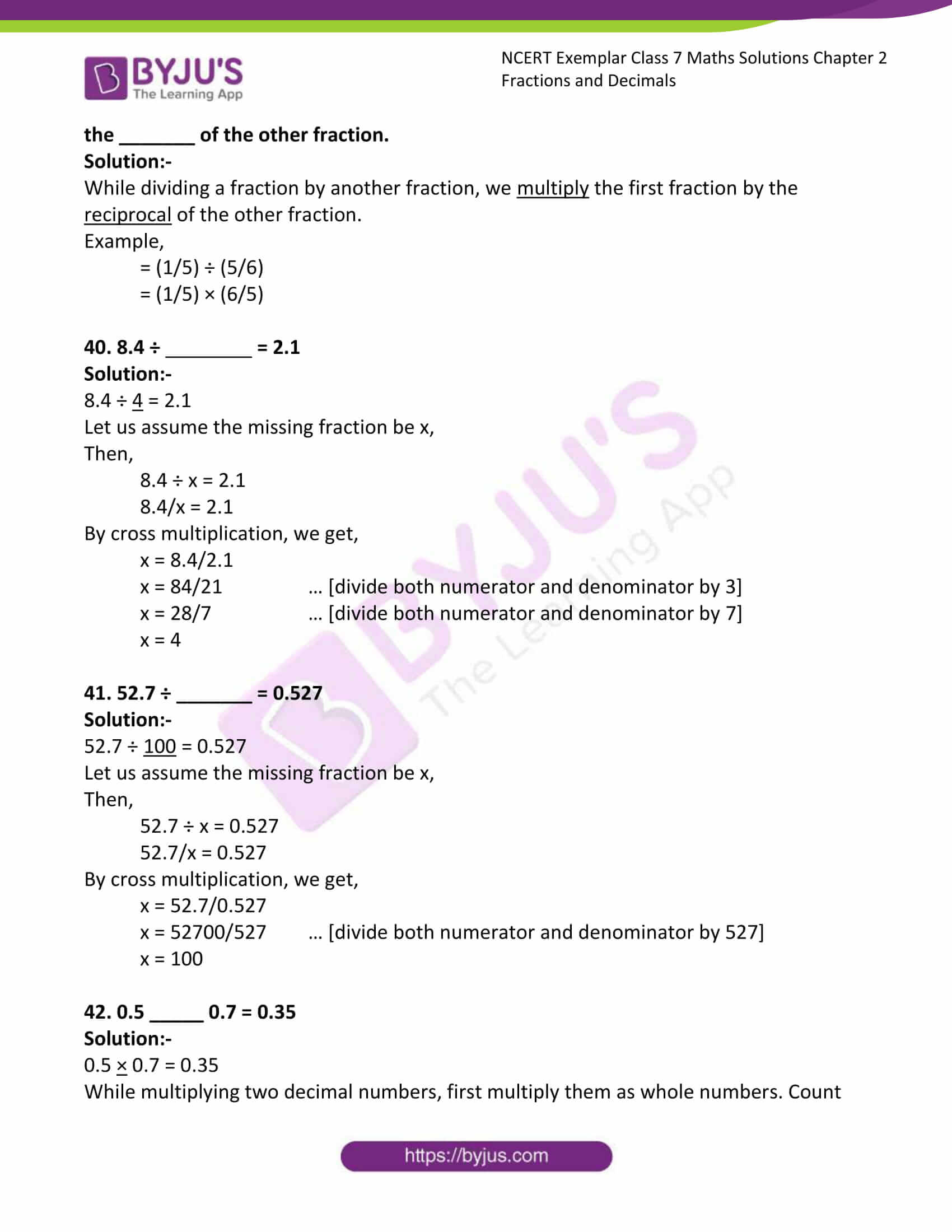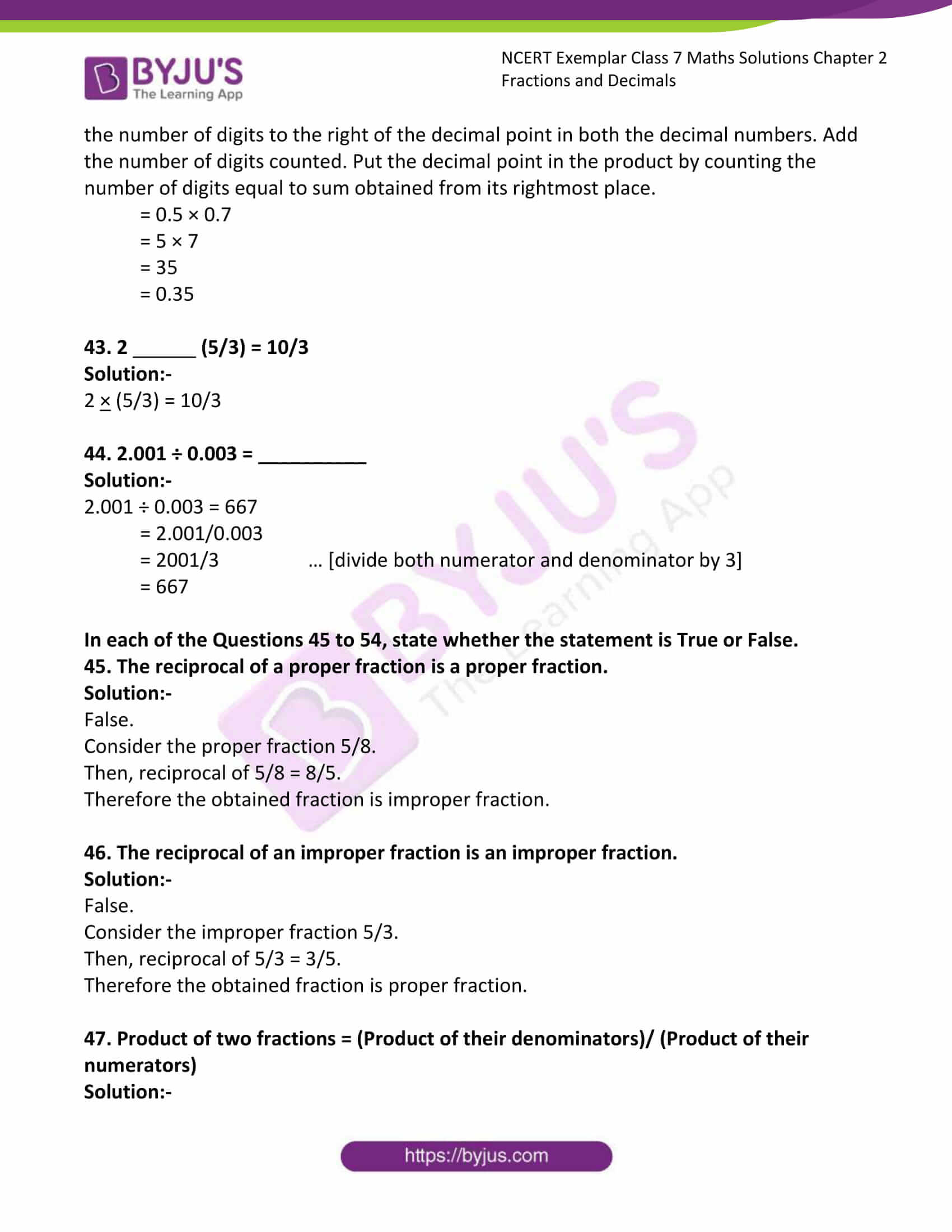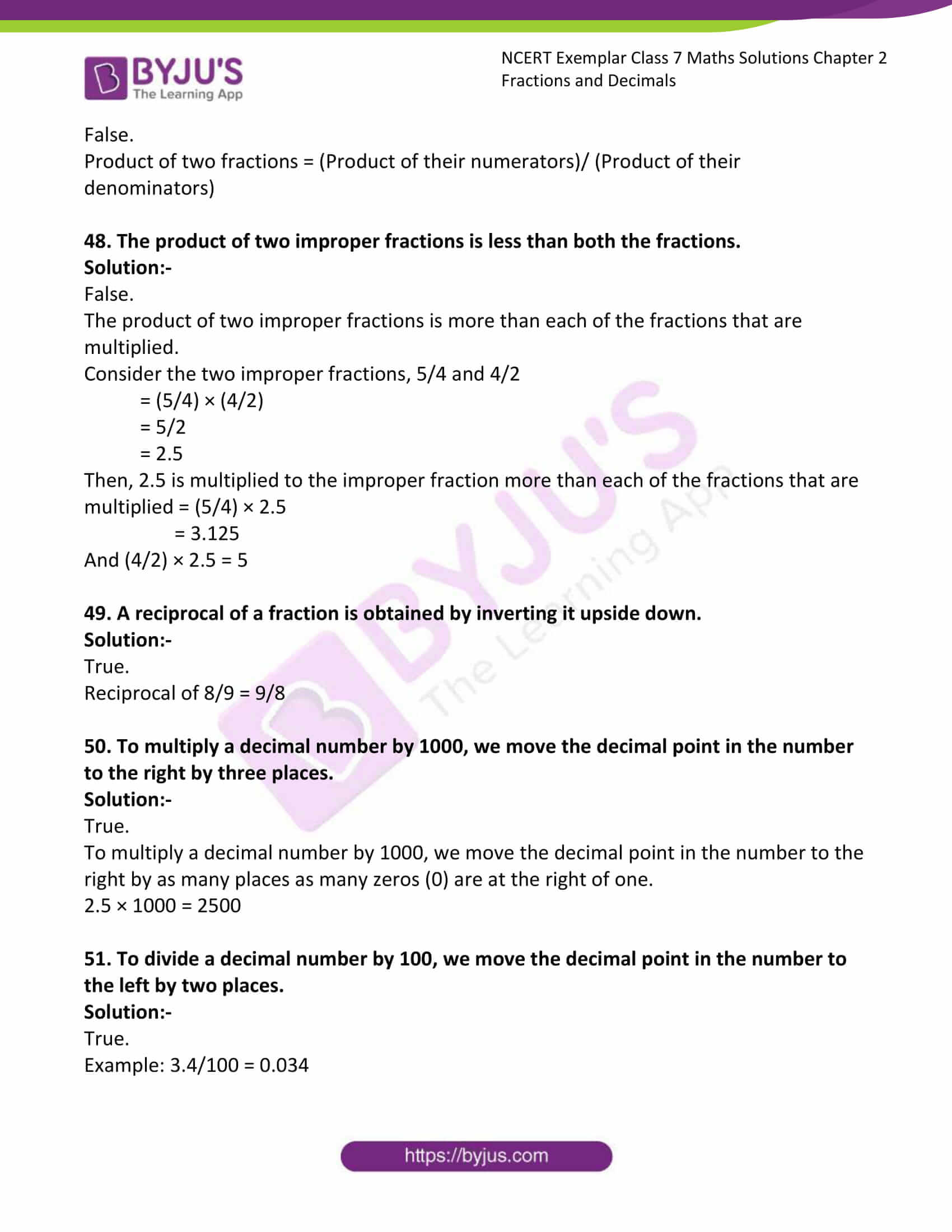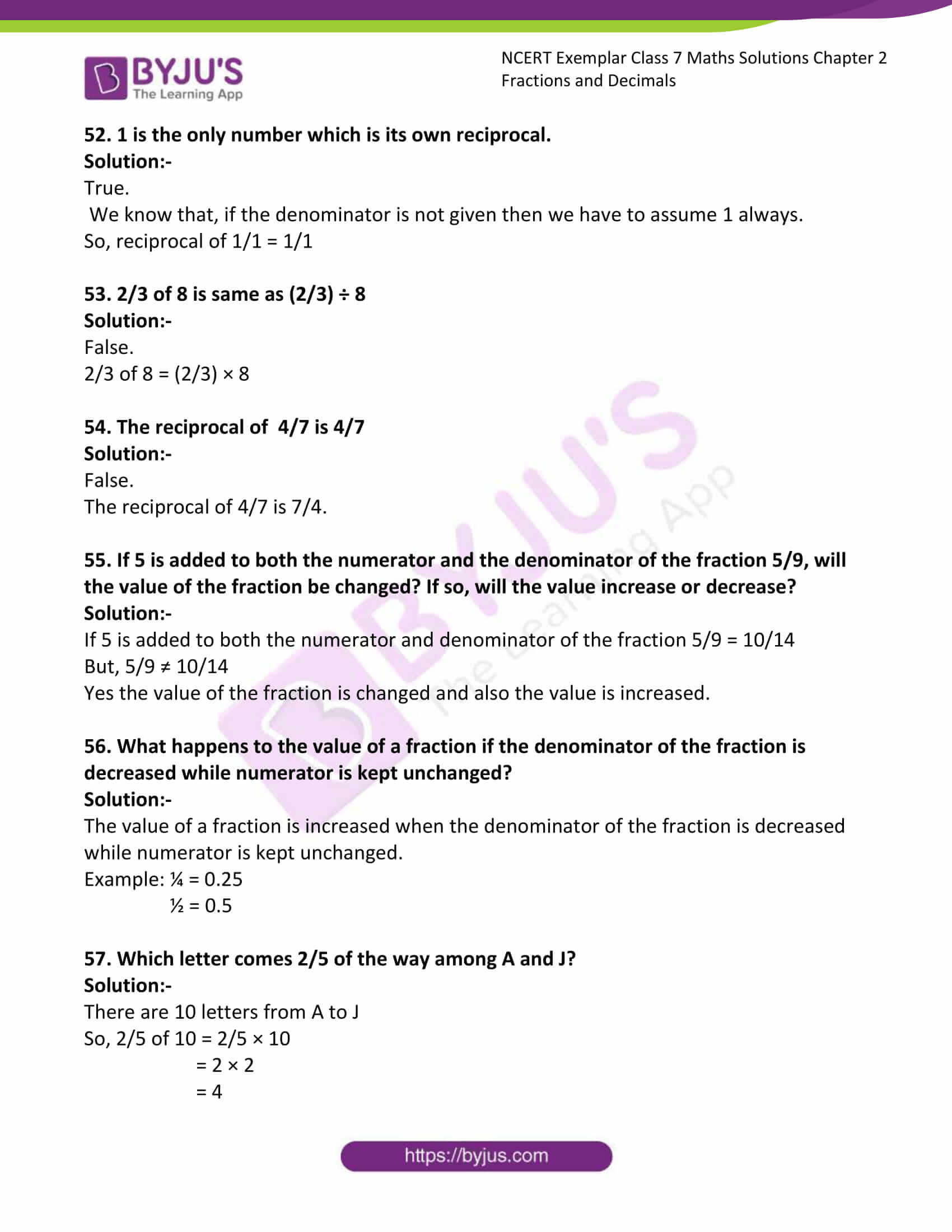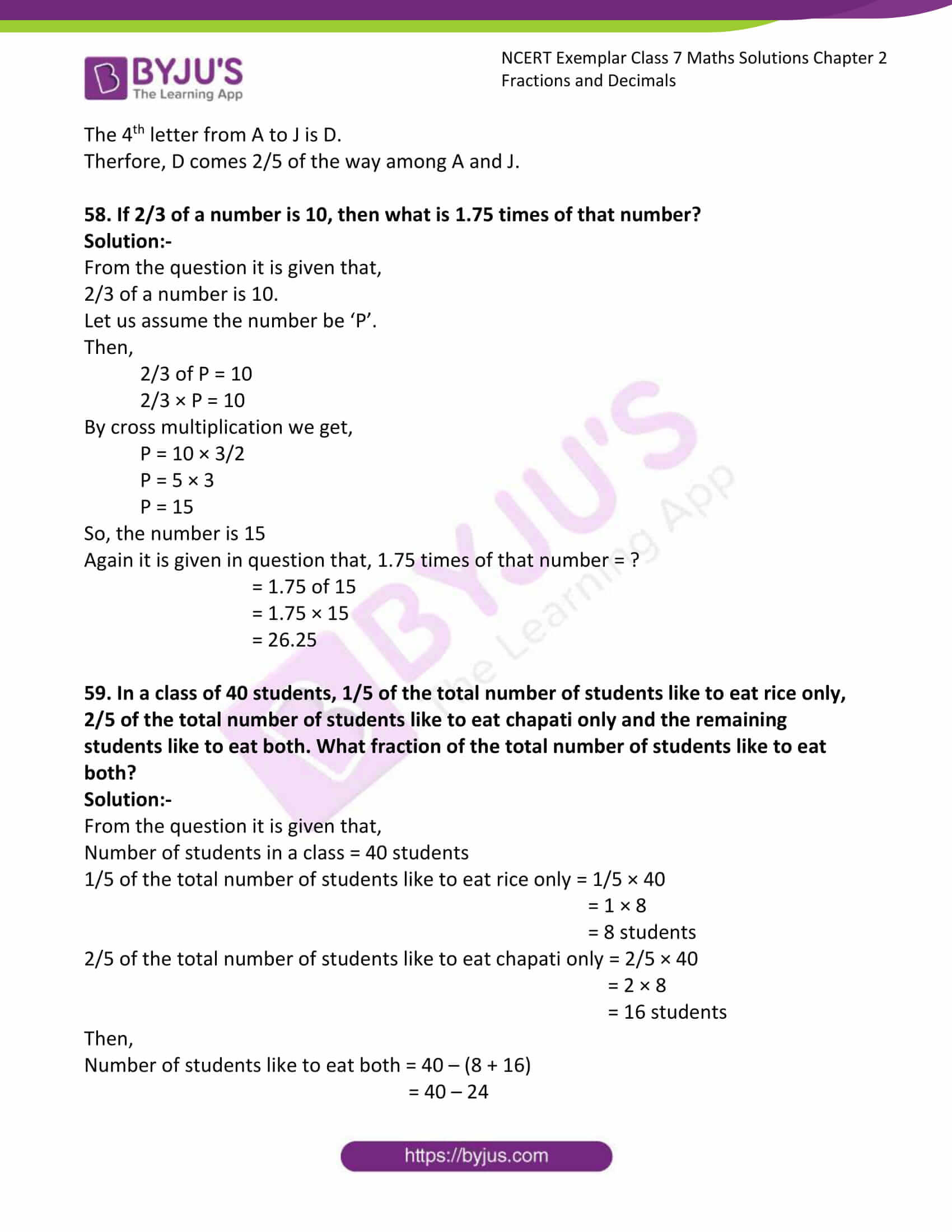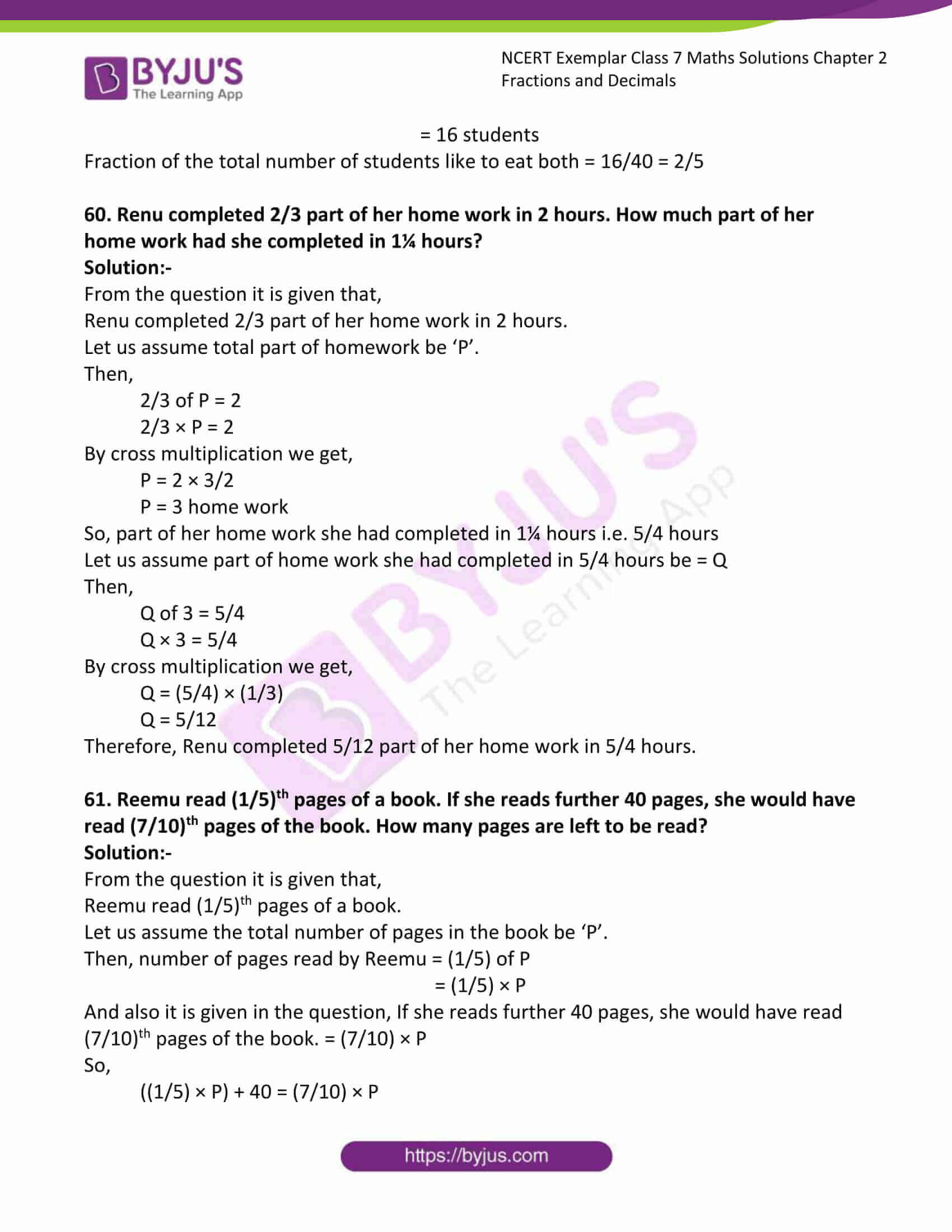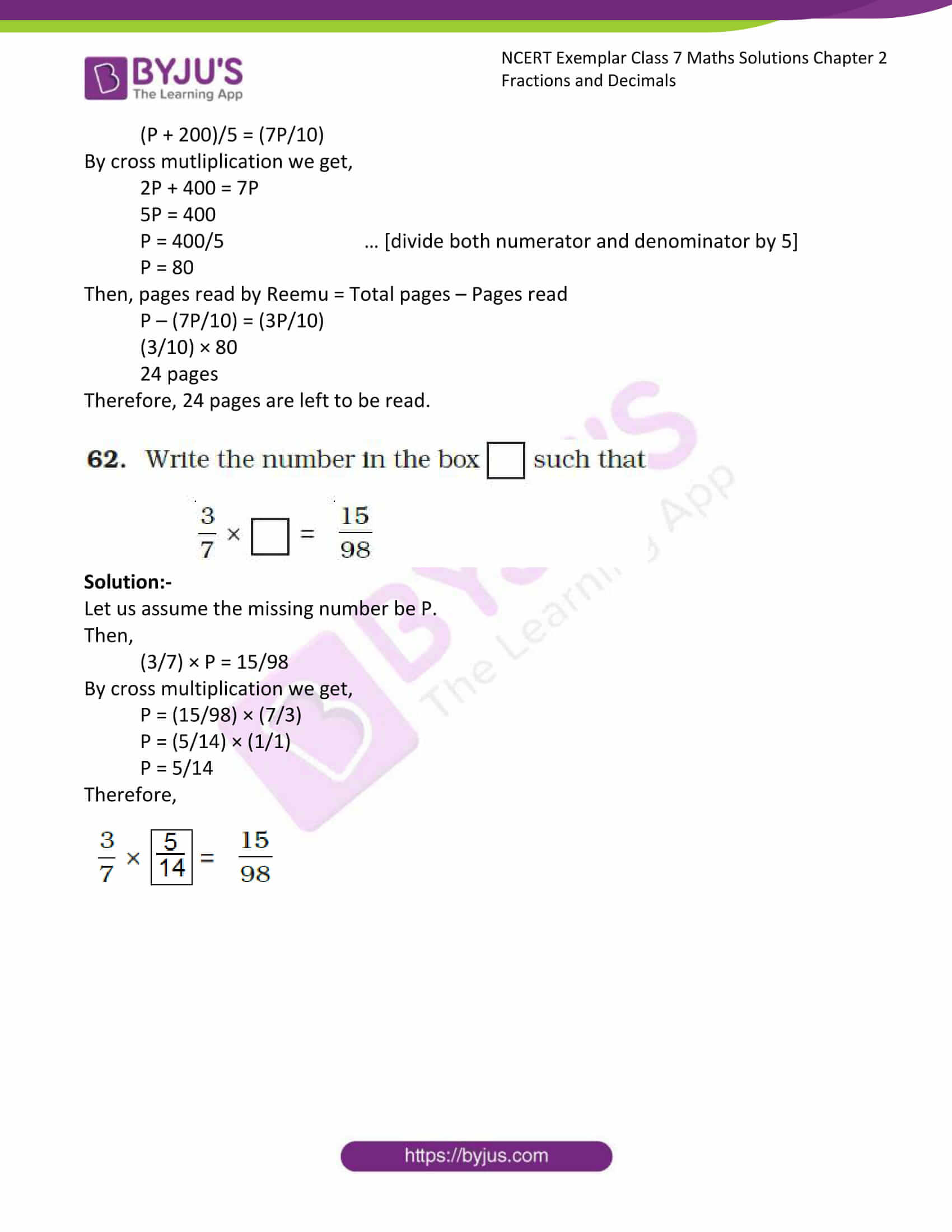### Access Answers to Maths NCERT Exemplar Solutions for Class 7 Chapter 2 Fractions and Decimals

Exercise Page: 38

In questions 1 to 20, out of four options, only one is correct. Write the correct answer.

1. (2/5) ×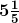is equal to:

(a) 26/25 (b) 52/25 (c) 2/5 (d) 6

Solution:-

(b) 52/25

First we have to convert the mixed fraction into improper fraction= 26/5

Then, (2/5) × (26/5)

= 52/25

2. 3¾ ÷ ¾ is equal to:

(a) 3 (b) 4 c) 5 (d) 45/16

Solution:-

c) 5

First we have to convert the mixed fraction into improper fraction 3¾ = 15/4

Then, 15/4 ÷ ¾

= (15/4)/ (¾)

= (15/4) × (4/3)

= (15 × 4)/ (4 ×3)

= (5 × 1)/ (1 ×1)

= 5

3. A ribbon of length 5¼ m is cut into small pieces each of length ¾m. Number of pieces will be:

(a) 5 (b) 6 (c) 7 (d) 8

Solution:-

(c) 7

First we have to convert the mixed fraction into improper fraction 5¼ = 21/4

Then, 21/4 ÷ ¾

= (21/4)/ (¾)

= (21/4) × (4/3)

= (21 × 4)/ (4 × 3)

= (7 × 1)/ (1 × 1)

= 7

4. The ascending arrangement of (2/3), (6/7), (13/21) is:

(a) 6/7, 2/3, 13/21 (b) 13/21, 2/3, 6/7

(c) 6/7, 13/21, 2/3 (d) 2/3, 6/7, 13/21

Solution:-

(b) 13/21, 2/3, 6/7

LCM of 21, 3, 7 = 21

Now, let us change each of the given fraction into an equivalent fraction having 21 as the denominator.

[(13/21) × (1/1)] = (13/21)

[(2/3) × (7/7)] = (14/21)

[(6/7) × (3/3)] = (18/21)

Clearly,

(13/21) < (14/21) < (18/21)

Hence,

(13/21) < (2/3) < (6/7)

Hence, the given fractions in ascending order are (13/21), (2/3), (6/7)

5. Reciprocal of the fraction 2/3 is:

(a) 2 (b) 3 (c) 2/3 (d) 3/2

Solution:-

(d) 3/2

The reciprocal of a non-zero fraction is obtained by interchanging its numerator and denominator.

6. The product of 11/13 and 4 is: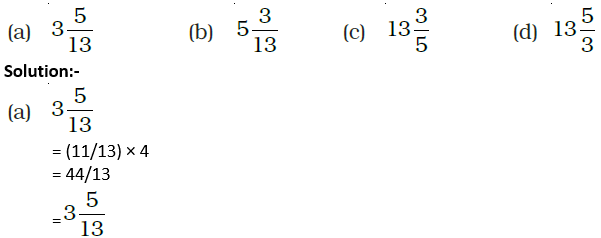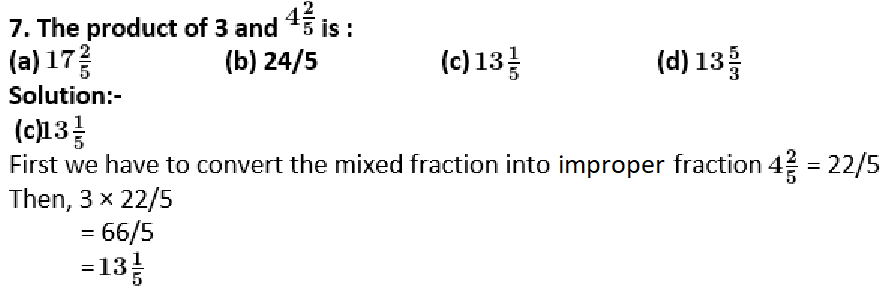8. Pictorial representation of 3 × 2/3 is: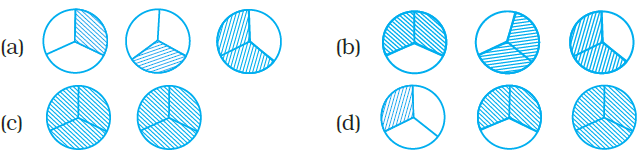Solution:-In the above figure, three circles are divided into 3 equal parts.

Out of 3 equal parts 2 equal parts are hatched.

9. 1/5 ÷ 4/5 equal to:

(a) 4/5 (b) 1/5 (c) 5/4 (d) ¼

Solution:-

(d) ¼

= 1/5 ÷ 4/5

= (1/5)/ (4/5)

= (1/5) × (5/4)

= 5/20 … [divide both numerator and denimonitor by 5]

= ¼

10. The product of 0.03 × 0.9 is:

(a) 2.7 (b) 0.27 (c) 0.027 (d) 0.0027

Solution:-

(c) 0.027

0.03 × 0.9 can be written as = (3/100) × (9/10)

= 27/1000

On dividing a decimal by 1000, the decimal point is shifted to the left by three places.

= 0.027

11. (5/7) ÷ 6 is equal to:

(a) 30/7 (b) 5/42 (c) 30/42 (d) 6/7

Solution:-

(b) 5/42

= 5/7 ÷ 6/1

= (5/7)/ (6/1)

= (5/7) × (1/6)

= 5/42

12.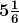÷ 9/2 is equal to:

(a) 31/6 (b) 1/27 (c)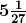(d) 31/27

Solution:-

(d) 31/27

First we have to convert the mixed fraction into improper fraction= 31/6

Then,

= 31/6 ÷ 9/2

= (31/6)/ (9/2)

= (31/6) × (2/9)

= (31/3) × (1/9)

= 31/27

13. Which of the following represents 1/3 of 1/6 ?

Solution:-

(a) (1/3) + (1/6) (b) (1/3) – (1/6)

(c) (1/3) × (1/6) (d) (1/3) ÷ (1/6)

Solution:-

(c) (1/3) × (1/6)

14. 3/7 of 2/5 is equal to

(a) 5/12 (b) 5/35 (c) 1/35 (d) 6/35

Solution:-

(d) 6/35

3/7 of 2/5 is equal to = (3/7) × (2/5)

= 6/35

15. One packet of biscuits requires 2½ cups of flour and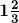cups of sugar. Estimated total quantity of both ingredients used in 10 such packets of biscuits will be

(a) less than 30 cups

(b) between 30 cups and 40 cups

(c) between 40 cups and 50 cups

(d) above 50 cups

Solution:-

(c) between 40 cups and 50 cups

From the question it is given that,

One packet of biscuits requires 2½ cups of flour = 5/2

One packet of biscuits requirescups of sugar. = 5/3

Total ingredients for one packet of biscuits = (5/2) + (5/3)

= (15 + 10)/6

= 25/6

Then, total quantity of both ingredients used in 10 such packets of biscuits = 10 × (25/6)

= 5 × (25/3)

= 125/3

=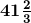16. The product of 7 and 6¾ is

(a) 42¼ (b) 47¼ (c) 42¾ (d) 47¾

Solution:-

(b) 47¼

First we have to convert the mixed fraction into improper fraction 6¾ = 27/4

= 7 × (27/4)

= 189/4

= 47¼

17. On dividing 7 by 2/5, the result is

(a) 14/2 (b) 35/4 (c) 14/5 (d) 35/2

Solution:-

(d) 35/2

= 7/ (2/5)

= 7 × (5/2)

= 35/2

18.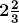÷ 5 is equal to

(a) 8/15 (b) 40/3 (c) 40/5 (d) 8/3

Solution:-

(a) 8/15

First we have to convert the mixed fraction into improper fraction= 8/3

Then,

= (8/3) ÷ 5

= (8/3)/(5/1)

= (8/3) × (1/5)

= 8/15

19. 4/5 of 5 kg apples were used on Monday. The next day 1/3 of what was left was used. Weight (in kg) of apples left now is

(a) 2/7 (b) 1/14 (c) 2/3 (d) 4/21

Solution:-

(c) 2/3

From the question it is given that,

4/5 of 5 kg apples were used on Monday = (4/5) × 5

= 20/5

= 4 kg

Then,

The next day 1/3 of what was left was used = (1/3) × 1

= 1/3 kg

So, Weight (in kg) of apples left now is = 1 – (1/3)

= (3 – 1)/3

= 2/3 kg of apples

20. The picture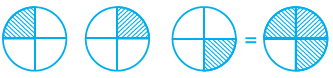Interprets

(a) ¼ ÷ 3 (b) 3 × ¼ (c) ¾ × 3 (d) 3 ÷ ¼

Solution:-

(b) 3 × ¼

From the given picture, ¼ + ¼ + ¼ = ¾

In Questions 21 to 44, fill in the blanks to make the statements true.

21. Rani ate 2/7 part of a cake while her brother Ravi ate 4/5 of the remaining. Part of the cake left is

Solution:-

Rani ate 2/7 part of a cake while her brother Ravi ate 4/5 of the remaining. Part of the cake left is 1/7.

Now, let us assume total part of cake be 1.

Then, from the question, given that Rani ate 2/7 part of a cake = 1 – (2/7)

= (7 – 2)/7

= 5/7

So, Ravi ate 4/5 of the remaining cake = (4/5) × (5/7)

= 4/7

Therefore, the part of the cake left = (5/7) – (4/7)

= 1/7

22. The reciprocal of 3/7 is

Solution:-

The reciprocal of 3/7 is 7/3

The reciprocal of a non-zero fraction is obtained by interchanging its numerator and denominator.

23. 2/3 of 27 is

Solution:-

2/3 of 27 is 18.

= 2/3 × 27

= 2 × 9

= 18

24. 4/5 of 45 is

Solution:-

4/5 of 45 is 36.

= 4/5 × 45

= 4 × 9

= 36

25. 4 ×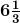is equal to

Solution:-

4 ×is equal to 76/3

First we have to convert the mixed fraction into improper fraction= 19/3

Then, 4 × 19/3

= 76/3

26. ½ of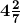is

Solution:-

½ ofis 15/7.

First we have to convert the mixed fraction into improper fraction= 30/7

Then, ½ × (30/7)

= 15/7

27. 1/9 of 6/5 is

Solution:-

1/9 of 6/5 is 2/15.

= (1/9) × (6/5)

= (1/3) × (2/5)

= 2/15

28. The lowest form of the product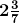× (7/9) is

Solution:-

The lowest form of the product× (7/9) is (17/9) or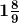First we have to convert the mixed fraction into improper fraction= 17/7

Then, (17/7) × (7/9)

= 17/9

=29. (4/5) ÷ 4 is equal to

Solution:-

(4/5) ÷ 4 is equal to 1/5.

= (4/5) ÷ 4

= (4/5) × (¼)

= (1/5) × (1/1)

= 1/5

30. 2/5 of 25 is

Solution:-

2/5 of 25 is 10

= 2/5 × 25

= 2 × 5

= 10

31. (1/5) ÷ (5/6) = (1/5) (6/5)

Solution:-

(1/5) ÷ (5/6) = (1/5) × (6/5)

While dividing one fraction by another fraction, we multiply the first fraction by the reciprocal of the other.

32. 3.2 × 10 = _______

Solution:-

3.2 × 10 = 32

To multiply a decimal number by 10, we move the decimal point in the number to the right by as many places as many zeros (0) are at the right of one.

33. 25.4 × 1000 = _______

Solution:-

25.4 × 1000 = 25400

To multiply a decimal number by 1000, we move the decimal point in the number to the right by as many places as many zeros (0) are at the right of one.

34. 93.5 × 100 = _______

Solution:-

93.5 × 100 = 9350

To multiply a decimal number by 100, we move the decimal point in the number to the right by as many places as many zeros (0) are at the right of one.

35. 4.7 ÷ 10 = ______

Solution:-

4.7 ÷ 10 = 0.47

To divide a decimal number by 10, shift the decimal point in the decimal number to the left by as many places as there are zeros over 1, to get the quotient.

= 4.7/10

= 0.47

36. 4.7 ÷ 100 = _____

Solution:-

4.7 ÷ 100 = 0.047

To divide a decimal number by 100, shift the decimal point in the decimal number to the left by as many places as there are zeros over 1, to get the quotient.

= 4.7/100

= 0.047

37. 4.7 ÷ 1000 = ______

Solution:-

4.7 ÷ 1000 = 0.0047

To divide a decimal number by 1000, shift the decimal point in the decimal number to the left by as many places as there are zeros over 1, to get the quotient.

= 4.7/1000

= 0.0047

38. The product of two proper fractions is _______ than each of the fractions that are multiplied.

Solution:-

The product of two proper fractions is less than each of the fractions that are multiplied.

Consider the two proper fractions, 4/5 and 2/4

= (4/5) × (2/4)

= 2/5

= 0.4

Then, 0.4 is multiplied to the proper fraction less than each of the fractions that are multiplied = (4/5) × 0.4

= 0.32

39. While dividing a fraction by another fraction, we _________ the first fraction by the _______ of the other fraction.

Solution:-

While dividing a fraction by another fraction, we multiply the first fraction by the reciprocal of the other fraction.

Example,

= (1/5) ÷ (5/6)

= (1/5) × (6/5)

40. 8.4 ÷ = 2.1

Solution:-

8.4 ÷ 4 = 2.1

Let us assume the missing fraction be x,

Then,

8.4 ÷ x = 2.1

8.4/x = 2.1

By cross multiplication, we get,

x = 8.4/2.1

x = 84/21 … [divide both numerator and denominator by 3]

x = 28/7 … [divide both numerator and denominator by 7]

x = 4

41. 52.7 ÷ _______ = 0.527

Solution:-

52.7 ÷ 100 = 0.527

Let us assume the missing fraction be x,

Then,

52.7 ÷ x = 0.527

52.7/x = 0.527

By cross multiplication, we get,

x = 52.7/0.527

x = 52700/527 … [divide both numerator and denominator by 527]

x = 100

42. 0.5 _____ 0.7 = 0.35

Solution:-

0.5 × 0.7 = 0.35

While multiplying two decimal numbers, first multiply them as whole numbers. Count the number of digits to the right of the decimal point in both the decimal numbers. Add the number of digits counted. Put the decimal point in the product by counting the number of digits equal to sum obtained from its rightmost place.

= 0.5 × 0.7

= 5 × 7

= 35

= 0.35

43. 2 (5/3) = 10/3

Solution:-

2 × (5/3) = 10/3

44. 2.001 ÷ 0.003 = __________

Solution:-

2.001 ÷ 0.003 = 667

= 2.001/0.003

= 2001/3 … [divide both numerator and denominator by 3]

= 667

In each of the Questions 45 to 54, state whether the statement is True or False.

45. The reciprocal of a proper fraction is a proper fraction.

Solution:-

False.

Consider the proper fraction 5/8.

Then, reciprocal of 5/8 = 8/5.

Therefore the obtained fraction is improper fraction.

46. The reciprocal of an improper fraction is an improper fraction.

Solution:-

False.

Consider the improper fraction 5/3.

Then, reciprocal of 5/3 = 3/5.

Therefore the obtained fraction is proper fraction.

47. Product of two fractions = (Product of their denominators)/ (Product of their numerators)

Solution:-

False.

Product of two fractions = (Product of their numerators)/ (Product of their denominators)

48. The product of two improper fractions is less than both the fractions.

Solution:-

False.

The product of two improper fractions is more than each of the fractions that are multiplied.

Consider the two improper fractions, 5/4 and 4/2

= (5/4) × (4/2)

= 5/2

= 2.5

Then, 2.5 is multiplied to the improper fraction more than each of the fractions that are multiplied = (5/4) × 2.5

= 3.125

And (4/2) × 2.5 = 5

49. A reciprocal of a fraction is obtained by inverting it upside down.

Solution:-

True.

Reciprocal of 8/9 = 9/8

50. To multiply a decimal number by 1000, we move the decimal point in the number to the right by three places.

Solution:-

True.

To multiply a decimal number by 1000, we move the decimal point in the number to the right by as many places as many zeros (0) are at the right of one.

2.5 × 1000 = 2500

51. To divide a decimal number by 100, we move the decimal point in the number to the left by two places.

Solution:-

True.

Example: 3.4/100 = 0.034

52. 1 is the only number which is its own reciprocal.

Solution:-

True.

We know that, if the denominator is not given then we have to assume 1 always.

So, reciprocal of 1/1 = 1/1

53. 2/3 of 8 is same as (2/3) ÷ 8

Solution:-

False.

2/3 of 8 = (2/3) × 8

54. The reciprocal of 4/7 is 4/7

Solution:-

False.

The reciprocal of 4/7 is 7/4.

55. If 5 is added to both the numerator and the denominator of the fraction 5/9, will the value of the fraction be changed? If so, will the value increase or decrease?

Solution:-

If 5 is added to both the numerator and denominator of the fraction 5/9 = 10/14

But, 5/9 ≠ 10/14

Yes the value of the fraction is changed and also the value is increased.

56. What happens to the value of a fraction if the denominator of the fraction is decreased while numerator is kept unchanged?

Solution:-

The value of a fraction is increased when the denominator of the fraction is decreased while numerator is kept unchanged.

Example: ¼ = 0.25

½ = 0.5

57. Which letter comes 2/5 of the way among A and J?

Solution:-

There are 10 letters from A to J

So, 2/5 of 10 = 2/5 × 10

= 2 × 2

= 4

The 4th letter from A to J is D.

Therfore, D comes 2/5 of the way among A and J.

58. If 2/3 of a number is 10, then what is 1.75 times of that number?

Solution:-

From the question it is given that,

2/3 of a number is 10.

Let us assume the number be ‘P’.

Then,

2/3 of P = 10

2/3 × P = 10

By cross multiplication we get,

P = 10 × 3/2

P = 5 × 3

P = 15

So, the number is 15

Again it is given in question that, 1.75 times of that number = ?

= 1.75 of 15

= 1.75 × 15

= 26.25

59. In a class of 40 students, 1/5 of the total number of students like to eat rice only, 2/5 of the total number of students like to eat chapati only and the remaining students like to eat both. What fraction of the total number of students like to eat both?

Solution:-

From the question it is given that,

Number of students in a class = 40 students

1/5 of the total number of students like to eat rice only = 1/5 × 40

= 1 × 8

= 8 students

2/5 of the total number of students like to eat chapati only = 2/5 × 40

= 2 × 8

= 16 students

Then,

Number of students like to eat both = 40 – (8 + 16)

= 40 – 24

= 16 students

Fraction of the total number of students like to eat both = 16/40 = 2/5

60. Renu completed 2/3 part of her home work in 2 hours. How much part of her home work had she completed in 1¼ hours?

Solution:-

From the question it is given that,

Renu completed 2/3 part of her home work in 2 hours.

Let us assume total part of homework be ‘P’.

Then,

2/3 of P = 2

2/3 × P = 2

By cross multiplication we get,

P = 2 × 3/2

P = 3 home work

So, part of her home work she had completed in 1¼ hours i.e. 5/4 hours

Let us assume part of home work she had completed in 5/4 hours be = Q

Then,

Q of 3 = 5/4

Q × 3 = 5/4

By cross multiplication we get,

Q = (5/4) × (1/3)

Q = 5/12

Therefore, Renu completed 5/12 part of her home work in 5/4 hours.

61. Reemu read (1/5)th pages of a book. If she reads further 40 pages, she would have read (7/10)th pages of the book. How many pages are left to be read?

Solution:-

From the question it is given that,

Reemu read (1/5)th pages of a book.

Let us assume the total number of pages in the book be ‘P’.

Then, number of pages read by Reemu = (1/5) of P

= (1/5) × P

And also it is given in the question, If she reads further 40 pages, she would have read (7/10)th pages of the book. = (7/10) × P

So,

((1/5) × P) + 40 = (7/10) × P

(P + 200)/5 = (7P/10)

By cross mutliplication we get,

2P + 400 = 7P

5P = 400

P = 400/5 … [divide both numerator and denominator by 5]

P = 80

P – (7P/10) = (3P/10)

(3/10) × 80

24 pages

Therefore, 24 pages are left to be read.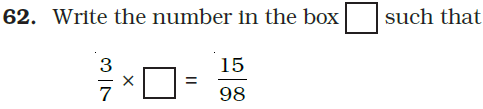Solution:-

Let us assume the missing number be P.

Then,

(3/7) × P = 15/98

By cross multiplication we get,

P = (15/98) × (7/3)

P = (5/14) × (1/1)

P = 5/14

Therefore,